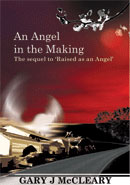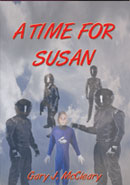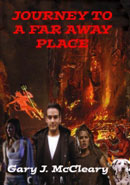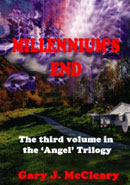# Free Book

AS a former Maths teacher I like to set mathematical problems. However they are at High School level and they are not too difficult.

Simply be the first to email me the correct answer to any mathematical problem below together with your name and address and I’ll send you a free signed copy of one of the books!

Problem 1

I have 210 meters of fencing wire and I wish to enclose a rectangular paddock on three sides with a natural boundary such as a river on the fourth side which does not need to be fenced. What should be the dimensions of the sides so as to enclose the maximum area and what is this maximum area?

Problem 2

I have \$1000 to invest at the current rate of 5.35% per year. If interest is added to the account at the end of each year how long will it take the account to reach \$10000?

Problem 3

For any circle the ratio of the circumference to the diameter is a number known as PI.
The first ten digits of PI are 3.141592654. What are the second twenty digits of PI, known as PI-2-20?

Problem 4

What is the sum of all the even numbers from 2 to 1000?

That is Sum = 2 + 4 +6 +8 +……………..+ 1000.

Problem 5

If men work at a constant rate and it takes 3 men 6 hours to dig 6 holes how long does it take 6 men to dig 4 holes?

Problem 6

In poker there are 4 suits of 13 cards each (23456789 10 J Q K A). A hand of five cards is called a ‘Full House’ if it consists of three of a kind and two of a kind. An example would be three aces and two kings. What is the probability of a poker hand of five cards being a ‘Full House’ ?

Problem 7

A river is 180 metres wide and the speed of the river current is 8 metres/second. There are two boats A and B each travelling at 10 m/s relative to the river. Boat A travels from point O on the bank to a point 180 m up stream against the current and then travels back down stream to point O with the current. Boat B travels across the current to a point 180 m away on the opposite bank and then back again to point O. (This boat obviously heads into the current both ways in order for the combination of its own speed and that of the current to enable it to reach a point on the opposite bank opposite to point O.) How long does it take each boat to complete its journey? Note that they are NOT the same.

NOTE: This led to one of the most important experiments in all of science namely the Michelson-Morley experiments conducted from 1881 to 1887. Instead of a boat travelling with and across a river it was a light ray travelling with and across the supposed ‘ether’. Despite the apparatus being sensitive enough NO difference in time has ever been recorded for the two light rays! This has far reaching effects and leads directly to Einstein’s Theory of Relativity. There is no ether and therefore no absolute frame of reference. The speed of light in a vacuum is always the same no matter how the observer moves!

Problem 8

Find P(not equal to 0) such that (X + 1/X)^2 + P^2 is a perfect square.This Site is Designed By Apex info Serve